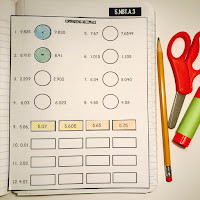Saturday, September 23, 2017

IMN Activities for Subtracting Decimals {Grade 5}

These interactive notebook activities are intended to help students understand how to read, write, and compare decimals to thousandths, compare two decimals to thousandths based on meanings of the digits in each place, using >, =, and < symbols to record the results of comparisons, and subtract decimals to hundredths, using concrete models or drawings and strategies based on place value, properties of operations.

Included are:
-4 different Subtracting Decimals activities to engage students
-Teacher facilitated activity for 60-90 minutes of classroom time
-Activities include: Vocabulary, Comparing and Ordering Decimals, Modeling subtracting decimals, and Practice lining up and subtracting decimals.
-Examples of Completed Interactive Math Notebook Activities are included.